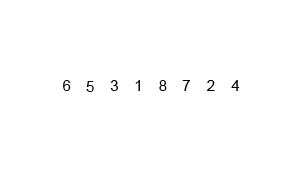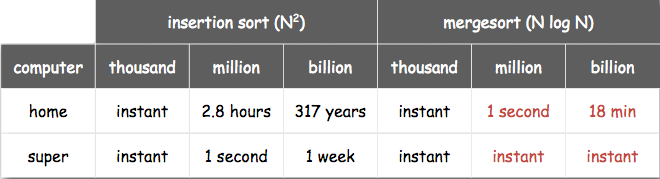After being developing software for a while, I realized that there is a couple of ways to become better at it. One it’s through your experience: writing code, working on projects, getting hands dirty… Other one it’s learning algorithms and design patterns. In other words through leveraging the experience of other computer scientists. Learning to use algorithms efficiently can instantly add to you the equivalent of 10 years of experience or more. Let’s get started and add new tools to our arsenal!

How do you know a piece of code that you just wrote is good enough? When you modify a program, how do you know if it is better as you found it? How do scale programs to handle huge amount of data? In fact, You cannot improve what you can’t measure.

How to measure them? We could count the number of seconds it takes to execute and compare it with other one. However, it’s not just troublesome to timers around code but if we run it in different hardware (e.g. supercomputer) it will seem like more efficient when indeed it’s exactly the same program. Let’s illustrate a better way with an example. Let’s say you want to sort an array of n integers.

## Sorting Algorithms

Do you recognize this algorithm? It’s called Insertion sort. It has two nested loops, which means that as the number of elements n in the array `arr` grows it will take approximately n * n longer to perform the sorting. In big-O notation, this will be represented like O(n^2). More on this notation later.

What would happen if the array arr is already sorted? That would be the best-case scenario. The inner for loop will never go through all the elements in the array then (because `arr[y-1] > arr[y]` won’t be met). So the algorithm in run in O(n).

We are not living in an ideal world. So O(n^2) will be probably the average time complexity. Can you think a better way of sorting an array of elements?

Take some minutes to think and come back…

### Merge Sort

A more efficient algorithm is the Merge sort. It uses the principle of divide and conquer to solve the problem faster. The idea is the follows:• Divide the array in half
• Divide the halves by half until 2 or 3 elements are remaining
• Sort each of these halves
• Merge them back together

Can you determine the time complexity of mergesort?

Even though the code is much longer, the algorithm is much more efficient.It would take some more knowledge to derive the running time mathematically, and we haven’t covered that yet. However, bear with me, it’s O(n log(n)). Let’s sum up:

Algorithm | best | average | worst | space complexity Insertion Sort | O(n) | O(n^2) | O(n^2) | O(1) Merge sort | O(n log(n)) | O(n log(n)) | O(n log(n)) | O(n)

Notice that the table has also the space complexity. How much space does the algorithms take is also an important parameter to compare algorithms. The merge sort uses an additional array that’s way its space complexity is `O(n)`, however, the insertion sort uses `O(1)` because it does the sorting in-place.

## Big O Notation

Big O is defined as the asymptotic upper limit of a function. In plain english, it means that is a function that cover the maximum values a function could take. As we saw a little earlier this notation help us to predict performance and compare algorithms.

Growth Rate Name
1 Constant
log(n) Logarithmic
n Linear
n log(n) Linearithmic
n^3 Cubic
2^n Exponential

This is kinda abstract let’s see what it means in code:

Growth Rate Name Code Example description
1 Constant
`a= b + 1;`
statement (one line of code)
log(n) Logarithmic
```      while(n>1){
n=n/2;
}
```
Divide in half (binary search)
n Linear
```for(c=0; c<n; c++){
a+=1;
}
```
Loop
n*log(n) Linearithmic Mergesort, Quicksort, ... Effective sorting algorithms
```for(c=0; c<n; c++){
for(i=0; i<n; i++){
a+=1;
}
}
```
Double loop
n^3 Cubic
```for(c=0; c<n; c++){
for(i=0; i<n; i++){
for(x=0; x<n; x++){
a+=1;
}
}
}
```
Triple loop
2^n Exponential Trying to braeak a password generating all possible combinations Exhaustive search

That’s all for this first part 1. I will continue publishing this tutorials every week or so. Stay tune!

**Update**

Checkout out the next post clicking here: Data Structures and Algorithms (DSA) for Beginners